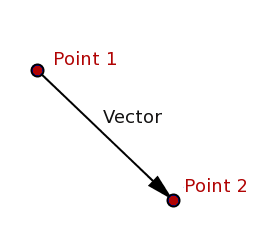#### Howdy, Stranger!

We are about to switch to a new forum software. Until then we have removed the registration on this forum.

# How would I be able to move a circle in different directions when the mouse is pressed?

edited March 2017

Hey I am creating a simple application to move the a circle in multiple direction when the mouse is pressed. For example, I clicked the lower left part of the circle, so then it would move up going to the right. Same as if I tap the ball on the lower right, it would move the ball going up to the left.

This is my code:

float ballX = 200; float ballY = 0; float h = 50;

float speedY = 2;

void setup() { // fullScreen();

size(400,400);

ellipseMode(CORNER);

}

void draw() { background(0); fill(255);

ellipse(ballX, ballY, h,h);

speedY = speedY + 0.5;

ballY = ballY + speedY;

if (ballY > height - h) { ballY = height - h; // speedY = speedY * -0.9; }

if (ballY <=0) { speedY = -speedY; } }

void mousePressed() { speedY=-10; }

Thanks for any help in advance :D

Tagged:

• Check if you clicked the ball. How far from the center of the ball does the click have to be for that to happen?

If the ball was clicked, where was the click? Where is the ball? What can you say about the difference between those positions? Using that, can you now update the ball's speed?

• @TfGuy44 Yes sir, that is what has been troubling me. I am wondering how would there be like a division on the ball so that when clicked on the particular division it would do the action sir.

How would I do that?

• Try this example: https://processing.org/examples/springs.html

You have a good code there for starters. What you need to do first is to check if you are inside the circle. Then you need to trace a vector from the mouse pointer to the center of the circle and that will direct the direction of motion. Are you familiar with vectors?

Kf

• @kfrajer Actualyl not yet sir, but your tutorial on springs is a good start and I might start reading up on Pvectors sir. :D

• @kfrajer Thank you soo much sir, this will be alot of help :D

• ``````float posx,posy,diameter=100;
void setup(){size(400,400);posx=posy=200;}
void draw(){background(180);
ellipse(posx,posy,diameter,diameter);}
void mousePressed(){
if(sq(mouseX-posx)+sq(mouseY-posy) < sq(diameter/2)){
posx = lerp(posx,mouseX,-0.2);
posy = lerp(posy,mouseY,-0.2);
}
}
``````
• Not that in your original code you have a speedY variable, but you don't have a speedX variable. @prince_polka's example works because it checks both the y (vertical) and x (horizontal).

You could also update and use the x and y pair as a PVector -- see for example the bouncing ball example:

PVector also has some useful things built in -- for example, `sub()` could allow you to subtract the center point of the ball from the mouse click point. This would give you a new vector with the direction between the two points -- which you could then scale to make a new speed for the ball, heading in that direction.